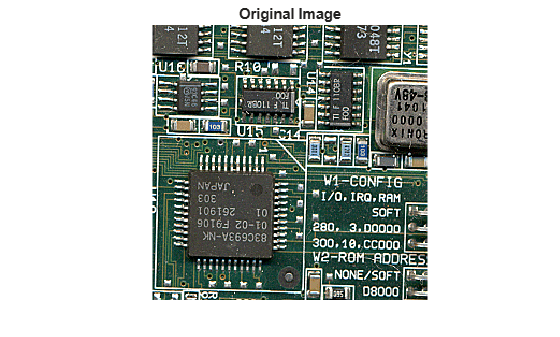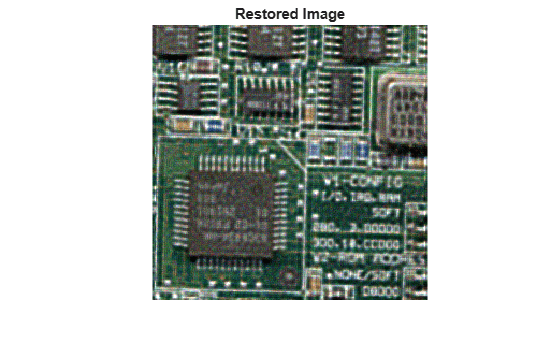# deconvlucy

Deblur image using Lucy-Richardson method

## Syntax

``J = deconvlucy(I,psf)``
``J = deconvlucy(I,psf,iter)``
``J = deconvlucy(I,psf,iter,dampar)``
``J = deconvlucy(I,psf,iter,dampar,weight)``
``J = deconvlucy(I,psf,iter,dampar,weight,readout)``
``J = deconvlucy(I,psf,iter,dampar,weight,readout,subsample)``

## Description

example

````J = deconvlucy(I,psf)` restores image `I` that was degraded by convolution with a point-spread function (PSF), `psf`, and possibly by additive noise. The algorithm is based on maximizing the likelihood that the resulting image `J` is an instance of the original image `I` under Poisson statistics.To improve the restoration, `deconvlucy` supports several optional parameters, described below. Use `[]` as a placeholder if you do not specify an intermediate parameter.```

example

````J = deconvlucy(I,psf,iter)` specifies the number of iterations, `iter`.```
````J = deconvlucy(I,psf,iter,dampar)` controls noise amplification by suppressing iterations for pixels that deviate a small amount compared to the noise, specified by the damping threshold `dampar`. By default, no damping occurs.```
````J = deconvlucy(I,psf,iter,dampar,weight)` specifies which pixels in the input image `I` are considered in the restoration. The value of an element in the `weight` array determines how much the pixel at the corresponding position in the input image is considered. For example, to exclude a pixel from consideration, assign it a value of `0` in the `weight` array. You can adjust the weight value assigned to each pixel according to the amount of flat-field correction.```
````J = deconvlucy(I,psf,iter,dampar,weight,readout)` specifies the additive noise (such as background or foreground noise) and variance of the read-out camera noise, `readout`.```
````J = deconvlucy(I,psf,iter,dampar,weight,readout,subsample)` uses subsampling when the PSF is given on a grid that is `subsample` times finer than the image.```

## Examples

collapse all

Read and display a pristine image that does not have blur or noise. This example optionally crops the image to a size of 256-by-256 with the top-left (x,y) coordinate at (2,50).

```I = imread('board.tif'); I = imcrop(I,[2 50 255 255]); imshow(I) title('Original Image')```Create a PSF that represents a Gaussian blur with standard deviation 5 and filter of size 5-by-5.

`PSF = fspecial('gaussian',5,5);`

Simulate blur in the image.

`blurred = imfilter(I,PSF,'symmetric','conv');`

```V = 0.002; blurred_noisy = imnoise(blurred,'gaussian',0,V); imshow(blurred_noisy) title('Blurred and Noisy Image')```Use `deconvlucy` to restore the blurred and noisy image. Specify the PSF used to create the blur and decrease the number of iterations to 5.

```luc1 = deconvlucy(blurred_noisy,PSF,5); imshow(luc1) title('Restored Image')```Create a sample image and blur it.

```I = checkerboard(8); PSF = fspecial('gaussian',7,10); V = .0001; BlurredNoisy = imnoise(imfilter(I,PSF),'gaussian',0,V);```

Create a weight array and call deconvlucy using several optional parameters.

```WT = zeros(size(I)); WT(5:end-4,5:end-4) = 1; J1 = deconvlucy(BlurredNoisy,PSF); J2 = deconvlucy(BlurredNoisy,PSF,20,sqrt(V)); J3 = deconvlucy(BlurredNoisy,PSF,20,sqrt(V),WT);```

Display the results.

```subplot(221);imshow(BlurredNoisy); title('A = Blurred and Noisy'); subplot(222);imshow(J1); title('deconvlucy(A,PSF)'); subplot(223);imshow(J2); title('deconvlucy(A,PSF,NI,DP)'); subplot(224);imshow(J3); title('deconvlucy(A,PSF,NI,DP,WT)');```## Input Arguments

collapse all

Blurry image, specified as a numeric array of any dimension. You can also specify the image as a cell array to enable interrupted iterations. For more information, see Tips.

Data Types: `single` | `double` | `int16` | `uint8` | `uint16`

PSF, specified as a numeric array.

Data Types: `single` | `double` | `int16` | `uint8` | `uint16`

Number of iterations, specified as a positive integer.

Data Types: `double`

Threshold for damping, specified as a numeric scalar. Damping occurs for pixels whose deviation between iterations is less than the threshold. `dampar` has the same data type as `I`.

Weight value of each pixel, specified as a numeric array with values in the range [0, 1]. `weight` has the same size as the input image, `I`. By default, all elements in `weight` have the value `1`, so all pixels are considered equally in the restoration.

Data Types: `double`

Noise, specified as a numeric scalar or numeric array. The value of `readout` corresponds to the additive noise (such as noise from the foreground and background) and the variance of the read-out camera noise. `readout` has the same data type as `I`.

Subsampling, specified as a positive scalar.

Data Types: `double`

## Output Arguments

collapse all

Deblurred image, returned as a numeric array or a 1-by-4 cell array. `J` (or `J{1}` when `J` is a cell array) has the same data type as `I`. For more information about returning `J` as a cell array for interrupted iterations, see Tips.

## Tips

• You can use `deconvlucy` to perform a deconvolution that starts where a previous deconvolution stopped. To use this feature, pass the input image `I` as a cell array, `{I}`. When you do, the `deconvlucy` function returns the output image `J` as a cell array, which you can then pass as the input array into the next `deconvlucy` call. The output cell array `J` contains four elements:

`J{1}` contains `I`, the original image.

`J{2}` contains the result of the last iteration.

`J{3}` contains the result of the next-to-last iteration.

`J{4}` is an array generated by the iterative algorithm.

• The output image `J` could exhibit ringing introduced by the discrete Fourier transform used in the algorithm. To reduce the ringing, use `I = edgetaper(I,psf)` before calling `deconvlucy`.

• `deconvlucy` converts the PSF to `double` without normalization.

• `deconvlucy` may return values in the output image that are beyond the range of the input image.

 D.S.C. Biggs and M. Andrews, Acceleration of iterative image restoration algorithms, Applied Optics, Vol. 36, No. 8, 1997.

 R.J. Hanisch, R.L. White, and R.L. Gilliland, Deconvolutions of Hubble Space Telescope Images and Spectra, Deconvolution of Images and Spectra, Ed. P.A. Jansson, 2nd ed., Academic Press, CA, 1997.